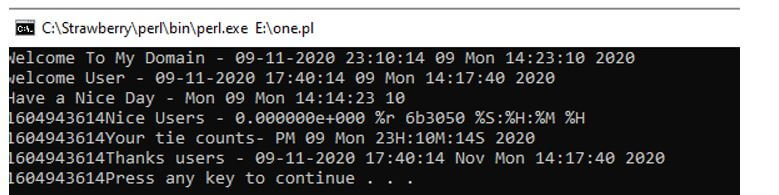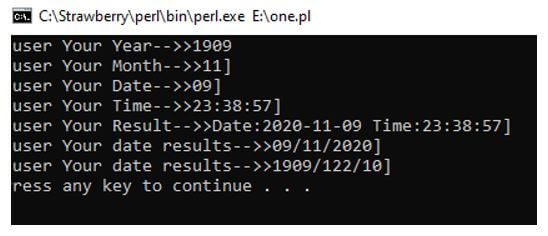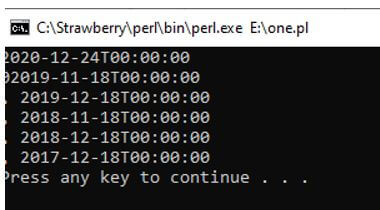# Perl datetime | How datetime Function Works in Perl with Examples

42## Introduction to Perl datetime

The Perl datetime is one of the features for the Perl scripting language using localtime() method; we want to calculate the current date and time of the machine there is no argument which is passed to the method for the date and time the method which handled by using the some predefined modules also using some import packages the default classes will used with their references according to the modules the date and time stamps will calculate more accuracy because when we calculate the time it depends upon the various units like milliseconds, seconds but the date will calculate and displayed with the same formats.

Syntax:

The Perl script always has the default variables, keywords, and functions used to handle the applications. Likewise, the datetime is the reference or instance of the specific function called localtime() this function which helps to display the current local date and time of the machine. Likewise, gmtime(), time() or the other predefined functions are calculated as the date and time intervals.

```#!/usr/bin/perl use DateTime; \$variable name = localtime(); \$var1 = DateTime -> now; ---some perl script codes based on the application requirements---```

The above codes are the basic syntax for creating the dates and times in the system with the help of localtime() function. The variables and other inbuilt methods are used for calculating the date and time intervals at the specific regions.

### How does the datetime Function work in Perl?

• The Perl script is most important, and it is widely used for portable and cross over platforms. It is mainly intended and co-ordinated with the other supporting platforms like object-oriented, stored procedurals etc. The Date and Time references are handled by using the pre-defined modules of the Perl scripts in that DateTime are one of the pre-defined modules, and using this module, the Perl script will use the default class as DateTime packages it ahs followed with some old and new scientific calendars with time formats. When we use localtime() method in Perl, the current date and time are shown; we won’t need any arguments to be passing in the method. Date Time is calculated using the some formats like %dd, %mm, %yy, %hh” date, month, year and hour when we call this reference in the script wherever it requires it. By using the “ set” keyword and methods the date and time are set for consolidated regions.
• If we want to set the “Africa” continent time zones, we can use the method called “set_time_zone(‘Africa’)” it will display the African continent time zones for all the areas. We also pass the argument with the specific areas of the continents like set_time_zone(“Africa/Johannesburg”) it will return the Africa continent with Johannesburg time it also been concatenated with the some datetime default methods like days, the previous day of the month, last_day_of_month, additional days if we want we can add the additional days by using the add(days => numbers) method by using these we will add the n number of days with manually moreover we also subtract the time intervals using the subtract(formats(seconds, milliseconds etc.) => numbers).
• Likewise we have also used set keyword with different methods set_year(), set_month(), set_day(), set_hour(), set_minute(), set_second(), set_millisecond() and set_nanosecond() these are the some various frequent methods which are used by the date-time references. The DateTime also set the locale country-wise region codes for populating the date and time intervals. If we use the locale codes like en_USA, en_UK, en_Italian etc. these codes have performed the application by using the country-wise based on the time zones the time will be displayed like GMT(Greenwich Mean Time) it will calculate the UK, England, London these are the some Europe based continents time will be displayed when we use IST (Indian Standard Time) format it will display the Indian based times. By using the set keyword reference methods, we have allocated the date and times for the user mentioned formats on the application.

### Examples of Perl datetime

Given below are the examples of Perl datetime:

#### Example #1

Code:

```#!/usr/local/bin/perl use POSIX qw(strftime); \$var1 = strftime "%c %d %a %S:%H:%M %Y", localtime; printf("Welcome To My Domain - \$var1n"); \$var1 = strftime "%c %d %a %S:%H:%M %Y", gmtime; printf("welcome User - \$var1n"); \$var1 = strftime "%a %d %a %S:%S:%H %M", localtime; printf("Have a Nice Day - \$var1n"); print time(); \$var1 = strftime "%e %r %p %S:%H:%M %H", gmtime; printf("Nice Users - \$var1n"); print time(); \$var1 = strftime "%p %d %a %HH:%MM:%SS %Y", localtime; printf("Your tie counts- \$var1n"); print time(); \$var1 = strftime "%c %b %a %S:%H:%M %Y", gmtime; printf("Thanks users - \$var1n"); print time();```

Output:#### Example #2

Code:

```use POSIX; use Time::Piece; my \$var = localtime(); my \$var1 = \$var->strftime('<Welcome User Your DateTimeFormats>'); use POSIX; use Time::Piece; my \$var = localtime(); my \$mn = \$var->strftime('%m'); my \$yr = \$var->strftime('%Y'); my \$dt = \$var->strftime('%d'); my \$tim = \$var->strftime('%X'); my \$rslt = \$var->strftime('Date:%Y-%m-%d Time:%X'); my \$d1 = localtime->dmy('/'); my (undef,undef,undef,\$yr,\$dyays,\$mnths) = localtime; \$yr = \$yr+1900; \$mnths += 2; if (length(\$mnths)  == 1) {\$mnths = "\$mnths";} if (length(\$yr) == 1) {\$yr = "\$yr";} my \$tdy = "\$yr/\$mnths/\$dyays"; print "[user Your Year-->>\$yrn"; print "[user Your Month-->>\$mn]n"; print "[user Your Date-->>\$dt]n"; print "[user Your Time-->>\$tim]n"; print "[user Your Result-->>\$rslt]n"; print "[user Your date results-->>\$d1]n"; print "[user Your date results-->>\$tdy]n";```

Output:#### Example #3

Code:

```#!/usr/bin/perl -w use strict; use DateTime; my (\$var, \$var1, \$var2, \$var3, \$var4, \$var5, \$var6, @dates); \$var = DateTime->new(year => 2020, day => 18, month => 11); \$var1 = DateTime->new(year => 2020, day => 18, month => 12); \$var2 = DateTime->new(year => 2019, day => 18, month => 11); \$var3 = DateTime->new(year => 2019, day => 18, month => 12); \$var4 = DateTime->new(year => 2018, day => 18, month => 11); \$var5 = DateTime->new(year => 2018, day => 18, month => 12); \$var6 = DateTime->new(year => 2017, day => 18, month => 11); \$var6 = DateTime->new(year => 2017, day => 18, month => 12); while (\$var <= \$var1) { if (\$var->day_of_week() > 6) { push(@dates, \$var); } \$var = \$var->add(days => 18); } for (@dates) { print \$_, \$/, \$-; print "\$var2n, \$var3n, \$var4n, \$var5n, \$var6n"; }```

Output:### Conclusion

In concluded part, the Perl datetime module will use the users for to customize the applications in the different regions. Due to the default methods, it can be achieved with the requirements the methods are achieving the overloading for both implicit and explicit conversions it has been used for all the inbuilt operations of the Perl scripts.

### Recommended Articles

This is a guide to Perl datetime. Here we discuss the introduction, how the datetime function works in Perl? Along with examples, respectively. You may also have a look at the following articles to learn more –

The post Perl datetime appeared first on EDUCBA.